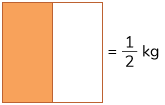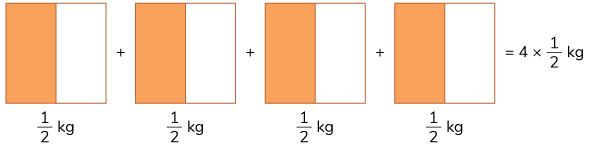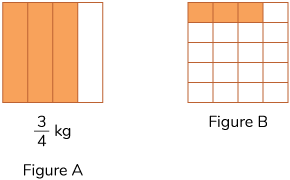# Multiplication of Fractions

Go back to  'Fractions'

## Multiplication, as a term, has been introduced in the early grades as “Repeated addition”. Adding the same value $$(v)$$ a certain number of times $$(n)$$ is the same as multiplying the value that many times $$(n \times v).$$ For instance, if there are $$3$$ friends and each of them has $$5$$ pens, how many pens do they have in total?

This problem can easily be solved by repeated addition. It can also be solved by multiplication. The only condition to be met is that the friends must all have the same number of pens. If each of them has different numbers of pens — say $$4,\;3,\;2,\;1$$ — their sum can be found through addition alone and not multiplication.

The same theory applies to the multiplication of fractions too! Let us see how.

## Multiplying a whole number by a fraction

What does \begin{align} 4 \times \frac{1}{2} \end{align} mean? It means that “there are $$4$$ packets of rice and each packet contains \begin{align} \frac{1}{2} \end{align} kg of rice.” How do we now find how much rice there is in total?

\begin{align} \frac{1}{2} \end{align} kg can be represented visually as given.As there are $$4$$ such packets that have \begin{align} \frac{1}{2} \end{align} kg rice, it can be represented as given,Rearranging this will give in,Alternately, we also know how to add fractions.

\begin{align} 4 \times \frac{1}{2} = \frac{1}{2} + \frac{1}{2} + \frac{1}{2} + \frac{1}{2} = \frac{1+1+1+1}{2} = \frac{4\; times \; 1}{2} \end{align}

It can also be written as \begin{align} 4 \times \frac{1}{2} = \frac{4 \times 1}{2} \end{align}

Multiplying a whole number $$(a)$$ with a fraction \begin{align} \frac{b}{c} \end{align} can be written as \begin{align} a \times \frac{b}{c} = \frac{(a \times b)}{c} \end{align}

Let us call this the “parallel-multiplication method”.

## Multiplying a fraction by another fraction

Let’s try and understand this using an example.

Example: How many kilograms of rice are there in \begin{align} \frac{1}{5} \end{align} packets of rice, if each packet has \begin{align} \frac{3}{4} \end{align} kg of rice?

Simply put, this can be written as \begin{align} \frac{1}{5} \times \frac{3}{4} \end{align}

Representing this visually would look like as given below,Each figure represents $$1$$ kg. Figure A represents \begin{align} \frac{3}{4} \end{align} kg and figure B represents \begin{align} \frac{1}{5} \end{align} of \begin{align} \frac{3}{4} \end{align} kg

How did we get Figure B? By dividing figure A into $$5$$ parts and then considering just one part of the five. Which means the answer is \begin{align} \frac{3}{20} \end{align} kg. The parallel-multiplication method also returns the same answer. In other words, the total number of parts is simply the product of the denominators, because our original $$4$$ parts (in Figure A) are each divided into $$5$$ parts each. The shaded part is the product of the numerators.

This gives us \begin{align} \frac{1}{5} \times \frac{3}{4} = \frac{(1 \times 3)}{(5 \times 4)} = \frac{3}{20} \end{align}

Therefore, \begin{align} \frac{a}{b} \times \frac{c}{d} = \frac{(a \times c)}{(b \times d)} \end{align}

## Multiplying a mixed/improper fraction by another fraction

Multiplying a mixed fraction would involve converting the mixed fraction into an improper one and then using the regular procedure.

Example: \begin{align} 1\frac{1}{5} \times \frac{3}{4} = \frac{6}{5} \times \frac{3}{4} = \frac{18}{20} \end{align}

## Tips and Tricks

• Generally, students simplify a fraction after multiplication. However to make calculations easier, check if the two fractions to be multiplied are already in the lowest form. If not, first simplify them and then multiply. Your calculation will be easier and the answer will not change.
E.g. \begin{align} \frac{4}{12} \times \frac{5}{13} \end{align} will be difficult to directly multiply. But simplifying the first fraction we get \begin{align} \frac{1}{3} \times \frac{5}{13} = \frac{5}{39}. \end{align}
• Simplification can also be done across the two fractions. E.g. if there is a common factor between the numerator of one of the fractions and the denominator of the other fraction, you can simplify them and proceed.
E.g. \begin{align} \frac{5}{28} \times \frac{7}{9} \end{align} can be simplified to \begin{align} \frac{5}{4} \times \frac{1}{9} \end{align} before multiplying.

### Common mistakes or misconceptions

• Children often tend to find the least common multiple of the denominators and multiply the numerators. This happens because they are incorrectly applying a recently learnt procedure of addition and subtraction of fractions to multiplication as well.
• A common misconception is that the product of a whole number and a fraction, and/or the product of two fractions is always greater than either of them. This happens because of this idea carries through from multiplication of whole numbers. For whole numbers, except when $$0$$ or $$1$$ are involved, the product is always greater than the two numbers being multiplied. This does not hold for multiplication involving proper fractions. E.g. \begin{align} \frac{1}{2} \times \frac{1}{4} = \frac{1}{8}. \end{align} One-eighth is smaller than both \begin{align} \frac{1}{2} \end{align} and \begin{align} \frac{1}{4}. \end{align}

## Multiply

Q1.  \begin{align} \frac{2}{3} \times \frac{7}{5} \end{align}

Q2.  \begin{align} \frac{8}{3} \times \frac{1}{6} \end{align}

Q3.  \begin{align} \frac{5}{4} \times \frac{9}{2} \end{align}